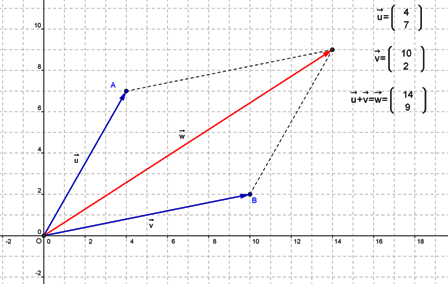A 2-dimensional vector can be represented as a pair of numbers (usually real numbers) such as $(a,b).$ Given two such vectors $(a,b)$ and $(c,d),$ the sum is obtained by adding the respective elements:

• $(a,b)~+~(c,d)~=~(a+c,b+d)$When representing the vectors as arrow on a plane, the corresponds to travelling the distance and direction represented by one vector, and then from there, continuing on in the direction of the second vector for the associated distance. This can be visualised as putting the vectors "head to tail."

Adding the vectors in the other order on the same diagram gives a parallelogram of vector addition, as shown in the diagram.

Related:

ArgandDiagram
ComplexNumber
MagnitudeOfAVector
MathematicsTaxonomy
MatrixMultiplication
Quaternion
ScalarProduct
SubtractingComplexNumbers
WhatIsATopic
(none) (none)
Vectors
VectorSubtraction

## You are here

RealNumber
ArgandDiagram
Associative
CategoryMetaTopic
CommonFactor
Commutative
ComplexNumber
ScalarProduct
(none) AlgebraicNumber
CauchySequence
ContinuedFraction
DedekindCut
IrrationalNumber
RationalNumber
TranscendentalNumber